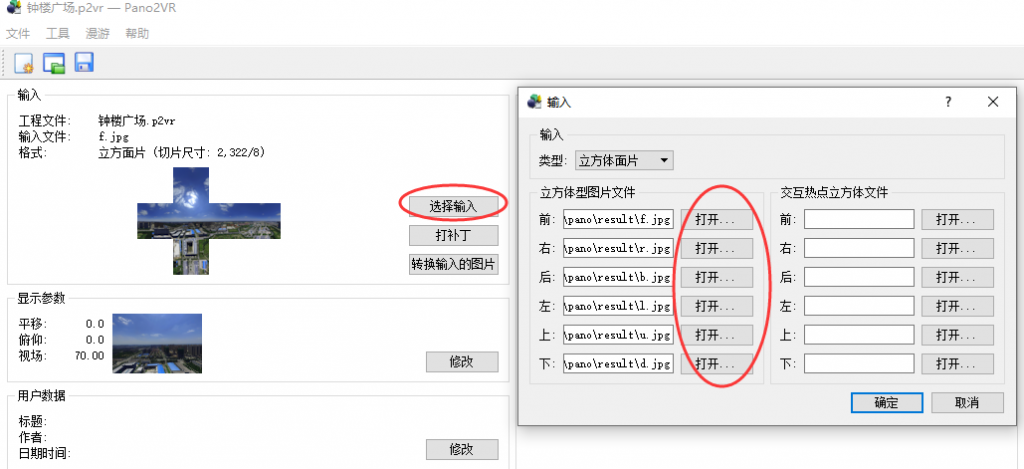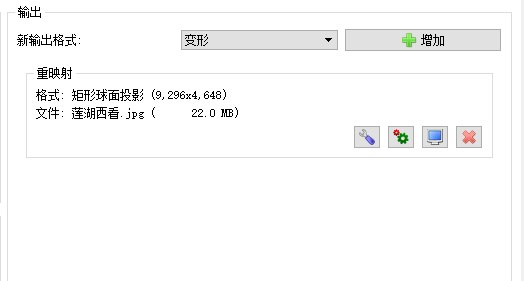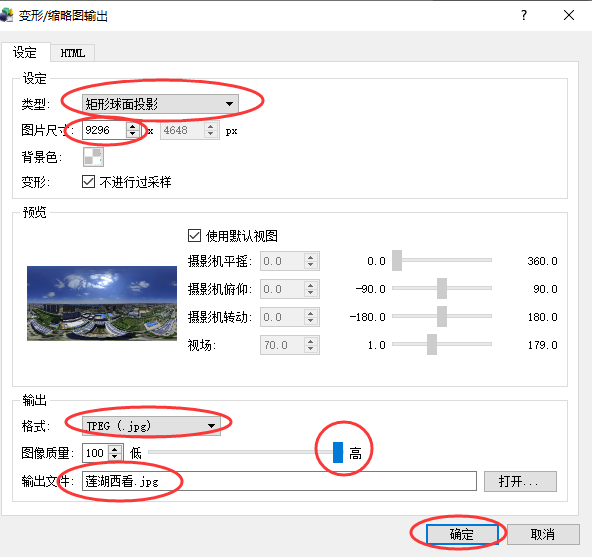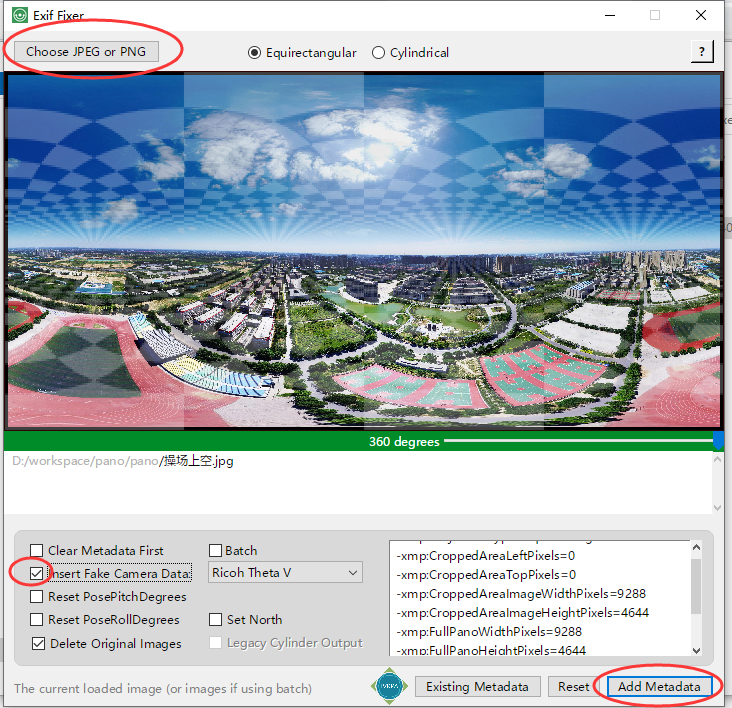# 下载720云的全景图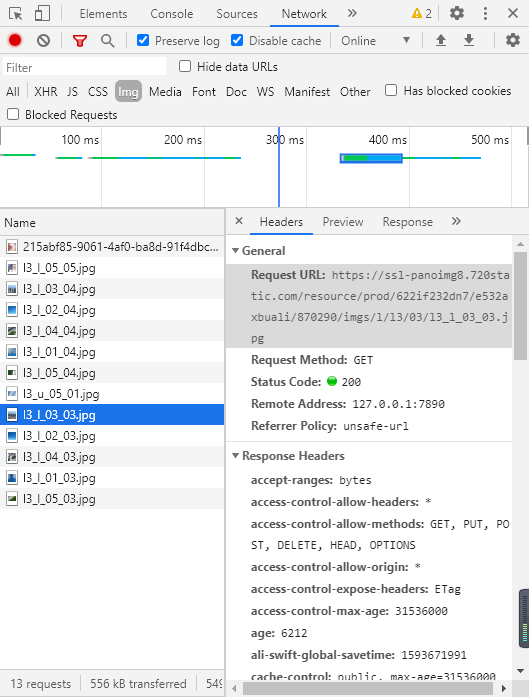l[1-3]_[f, b, l, r, u, d]_0[1-5]_0[1-5].jpg

# 构造6个面
for faceCode in ('b','d','f','l','r','u'):
# 似乎有3层清晰度，这里只取第三层
for layerCode in range(3,4):
# 每层里面一般又有5个子文件夹（表示矩阵的行）
for subFold in range(1,6):
# 每个子文件夹里面又有5个图片（表示矩阵的列）
for picCode in range(1,6):
pathStr = "{}/l{}/0{}/l{}_{}_0{}_0{}.jpg".format(faceCode, layerCode, subFold, layerCode, faceCode, subFold, picCode)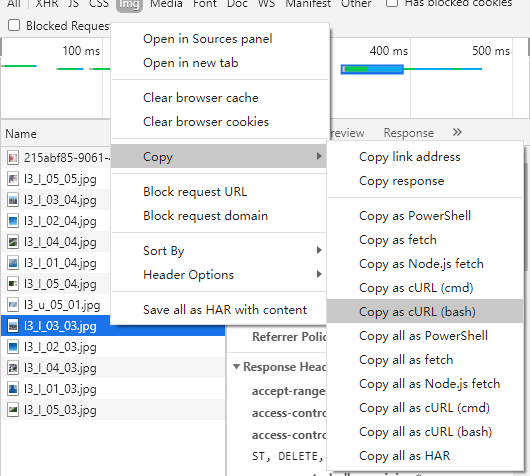headers = {
'Content-Type': 'image/jpeg',
'authority': 'ssl-panoimg6.720static.com',
'pragma': 'no-cache',
'cache-control': 'no-cache',
'origin': 'https://720yun.com',
'user-agent': 'Mozilla/5.0 (Windows NT 10.0; Win64; x64) AppleWebKit/537.36 (KHTML, like Gecko) Chrome/83.0.4103.116 Safari/537.36',
'dnt': '1',
'accept': 'image/webp,image/apng,image/*,*/*;q=0.8',
'sec-fetch-site': 'cross-site',
'sec-fetch-mode': 'cors',
'sec-fetch-dest': 'image',
'referer': originUrl,
'accept-language': 'zh-CN,zh;q=0.9,en-US;q=0.8,en;q=0.7'
}


for i in range(len(urlsList)):
url = urlsList[i]
print('\r'*8 + "{}/{}".format(i+1, len(urlsList)), end='')
try:
except Exception as e:
print('get 出了点问题：{}'.format(e))

if r.status_code == 200:
with open('img/' + url.split('/')[-1], 'wb') as f:
f.write(r.content)

print("\n下载完成")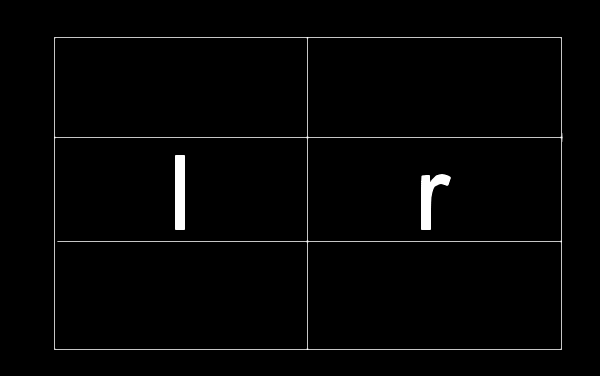# together.py
import PIL
from PIL import Image

def 横向拼接(imagesList):
# 检查图像高度是否一致
# 方法是生成高度列表并和另一个内部元素完全是和第一个一样的列表相比较
heights = list(map(lambda image: image.size, imagesList))
isHeightEqual = [heights for x in range(len(imagesList))] == heights
if not isHeightEqual:
raise Exception('高度不匹配')

widths = tuple(map(lambda image: image.size, imagesList))
# 新建画布
imageBig = Image.new(mode='RGB', size = (sum(widths), heights))
# 开始逐个粘贴
for i in range(len(imagesList)):
image  = imagesList[i]
imageBig.paste(image, (sum(widths[:i]), 0, sum(widths[:i+1]), image.size))
return imageBig

def 竖向拼接(imagesList):
# 检查图像宽度是否一致
widths = list(map(lambda image: image.size, imagesList))
isWidthsEqual = [widths for x in range(len(imagesList))] == widths
if not isWidthsEqual:
raise Exception('宽度不匹配')

heights = tuple(map(lambda image: image.size, imagesList))
# 新建画布
imageBig = Image.new( mode='RGB', size = (widths, sum(heights)) )
# 开始逐个粘贴
for i in range(len(imagesList)):
image  = imagesList[i]
imageBig.paste(image, (0, sum(heights[:i]), image.size, sum(heights[:i+1])))

return imageBig

# matrixD 矩阵维度元组
# flatImagesList 用于拼接的扁平化的图像列表
def 矩阵拼接(matrixD, flatImagesList):
if matrixD * matrixD != len(flatImagesList):
raise Exception("给定矩阵尺寸与得到的图像个数不符。")

imagesLines = list()
# 拼接所有行
for lineIndex in range(matrixD):
imagesLines.append(横向拼接(flatImagesList[lineIndex * matrixD : (lineIndex + 1) * matrixD]))

# 竖向拼接
return 竖向拼接(imagesLines)

def 拼接所有(path):
import os
import math
fileNames = os.listdir(path)

# 按文件名整理各个面的文件名到一个字典中
sixFaceDict = {}
for fileName in fileNames:
sixFaceDict[fileName] = sixFaceDict.get(fileName, list())
sixFaceDict[fileName].append(path + fileName)

# 按路径全部替换成image
for face in sixFaceDict:
for i in range(len(sixFaceDict[face])):
sixFaceDict[face][i] = Image.open(sixFaceDict[face][i])

# 拼接
for face in sixFaceDict:
linesNum = int(math.sqrt(len(sixFaceDict[face])))
sixFaceDict[face] = 矩阵拼接((linesNum, linesNum), sixFaceDict[face])

# 输出宽度
print("pano width should be: {}".format(
sixFaceDict['f'].size +
sixFaceDict['b'].size +
sixFaceDict['l'].size +
sixFaceDict['r'].size
))

# 保存
for face in sixFaceDict:
sixFaceDict[face].save('result/'+ face + '.jpg')

if __name__ == '__main__':
拼接所有( 'img/')


# dimg.py
# origin url e.g. https://720yun.com/t/3922fmO5jyg?scene_id=640863
# request url e.g. https://ssl-panoimg6.720static.com/resource/prod/622if232dn7/e532axbuali/521330/imgs/
originUrl = input('Please input origin url: ')
urlsList = list()

# 构造6个面
for faceCode in ('b','d','f','l','r','u'):
# 似乎有3层清晰度，这里只取第三层
for layerCode in range(3,4):
# 每层里面一般又有5个子文件夹（表示矩阵的行）
for subFold in range(1,6):
# 每个子文件夹里面又有5个图片（表示矩阵的列）
for picCode in range(1,6):
pathStr = "{}/l{}/0{}/l{}_{}_0{}_0{}.jpg".format(faceCode, layerCode, subFold, layerCode, faceCode, subFold, picCode)

# 开始请求
import requests

'Content-Type': 'image/jpeg',
'authority': 'ssl-panoimg6.720static.com',
'pragma': 'no-cache',
'cache-control': 'no-cache',
'origin': 'https://720yun.com',
'user-agent': 'Mozilla/5.0 (Windows NT 10.0; Win64; x64) AppleWebKit/537.36 (KHTML, like Gecko) Chrome/83.0.4103.116 Safari/537.36',
'dnt': '1',
'accept': 'image/webp,image/apng,image/*,*/*;q=0.8',
'sec-fetch-site': 'cross-site',
'sec-fetch-mode': 'cors',
'sec-fetch-dest': 'image',
'referer': originUrl,
'accept-language': 'zh-CN,zh;q=0.9,en-US;q=0.8,en;q=0.7'
}

for i in range(len(urlsList)):
url = urlsList[i]
print('\r'*8 + "{}/{}".format(i+1, len(urlsList)), end='')
try:
except Exception as e:
print('get 出了点问题：{}'.format(e))

if r.status_code == 200:
with open('img/' + url.split('/')[-1], 'wb') as f:
f.write(r.content)

print("\n下载完成")

import together
together.拼接所有('img/')